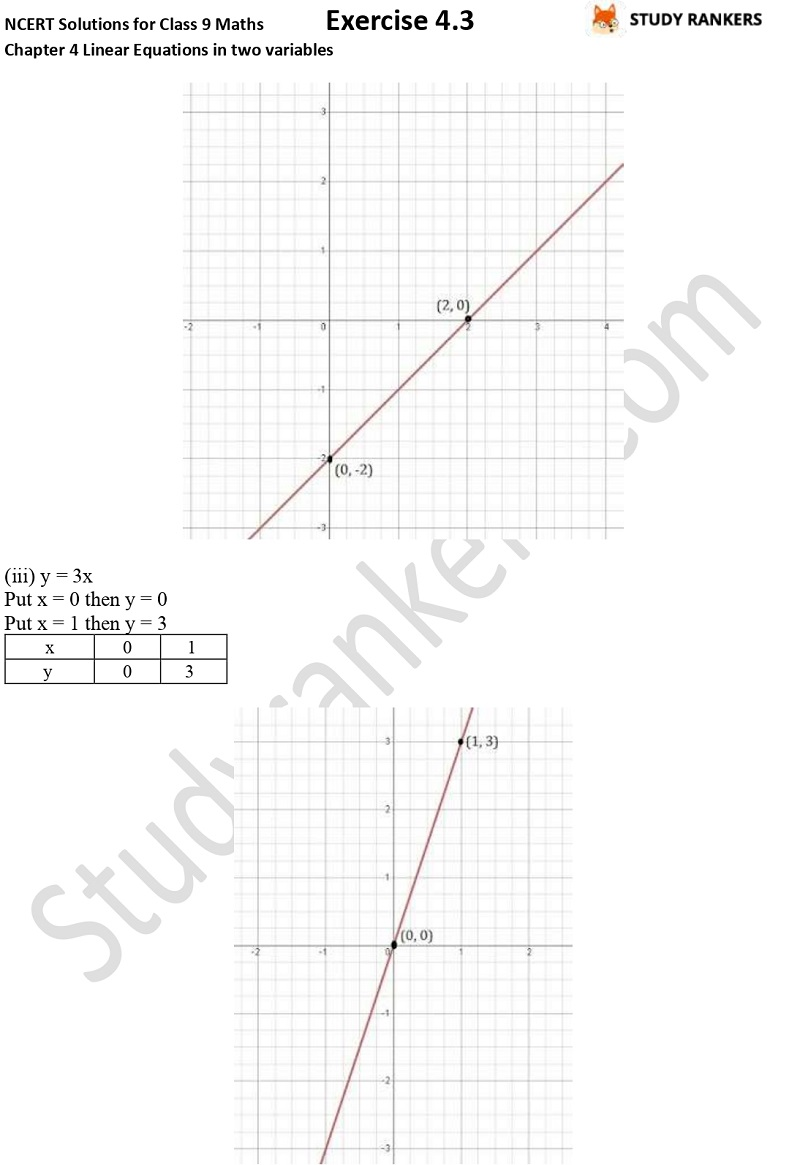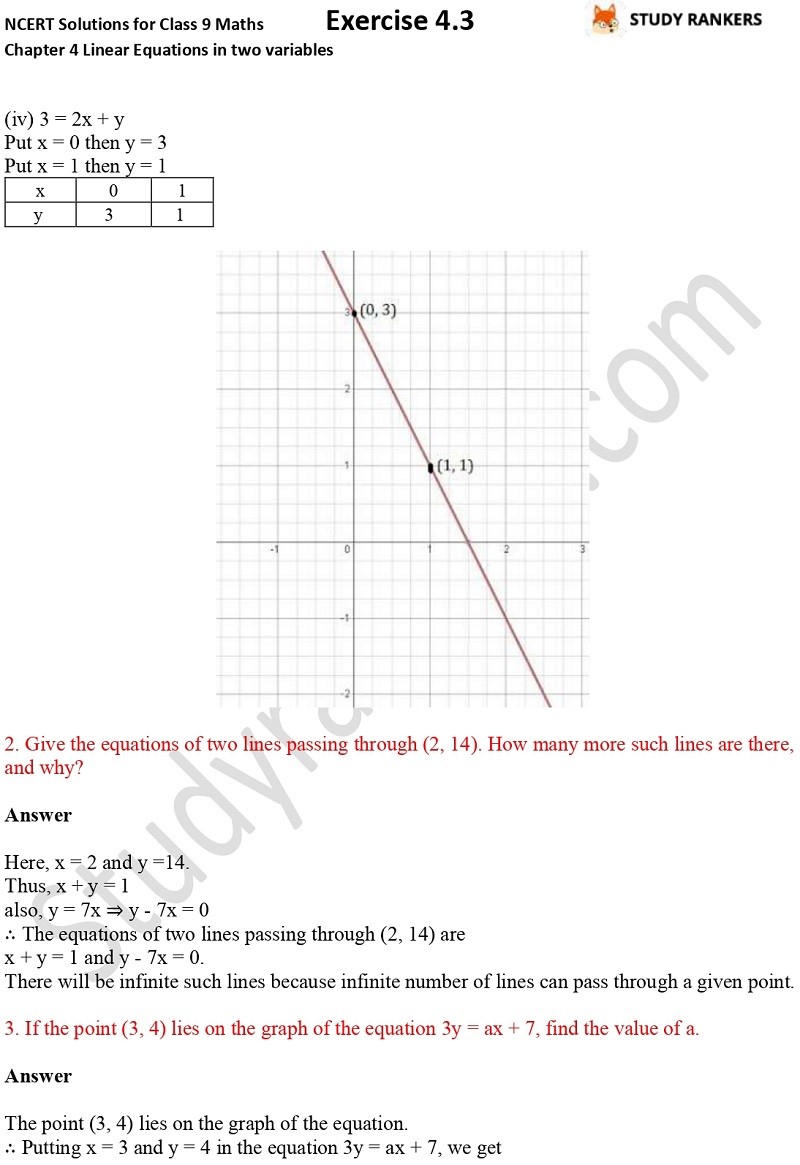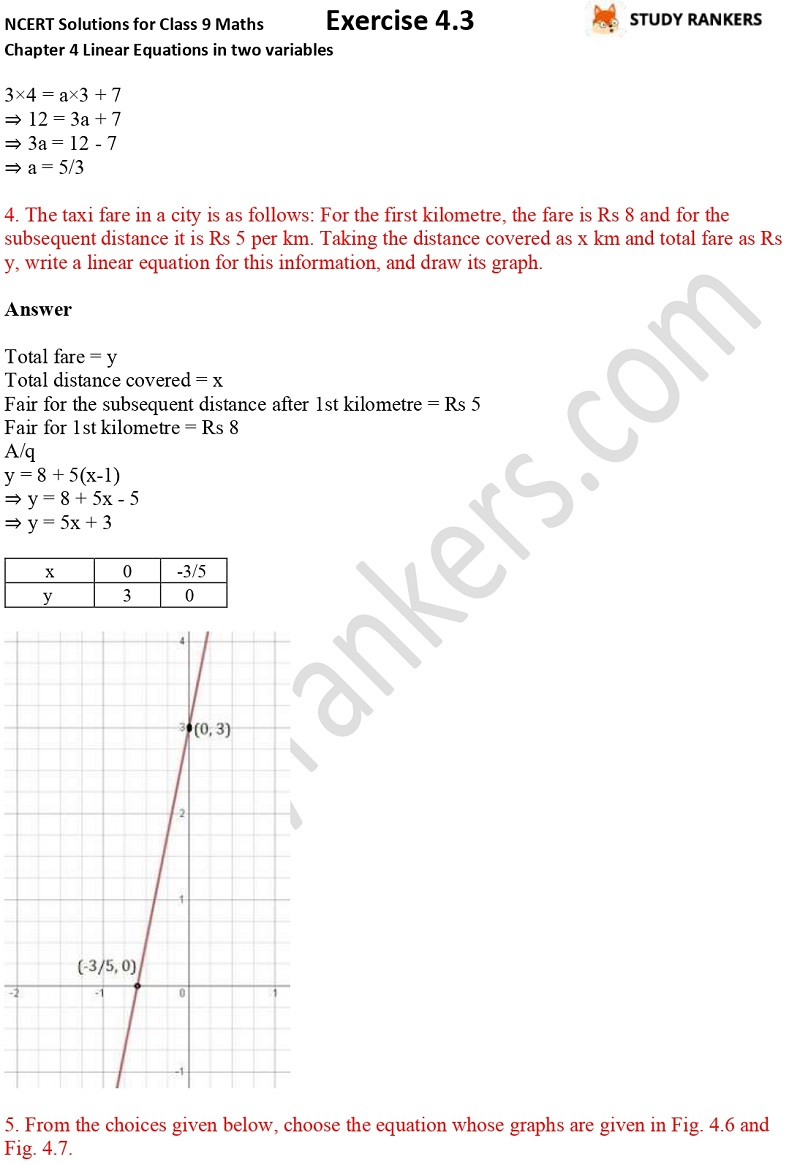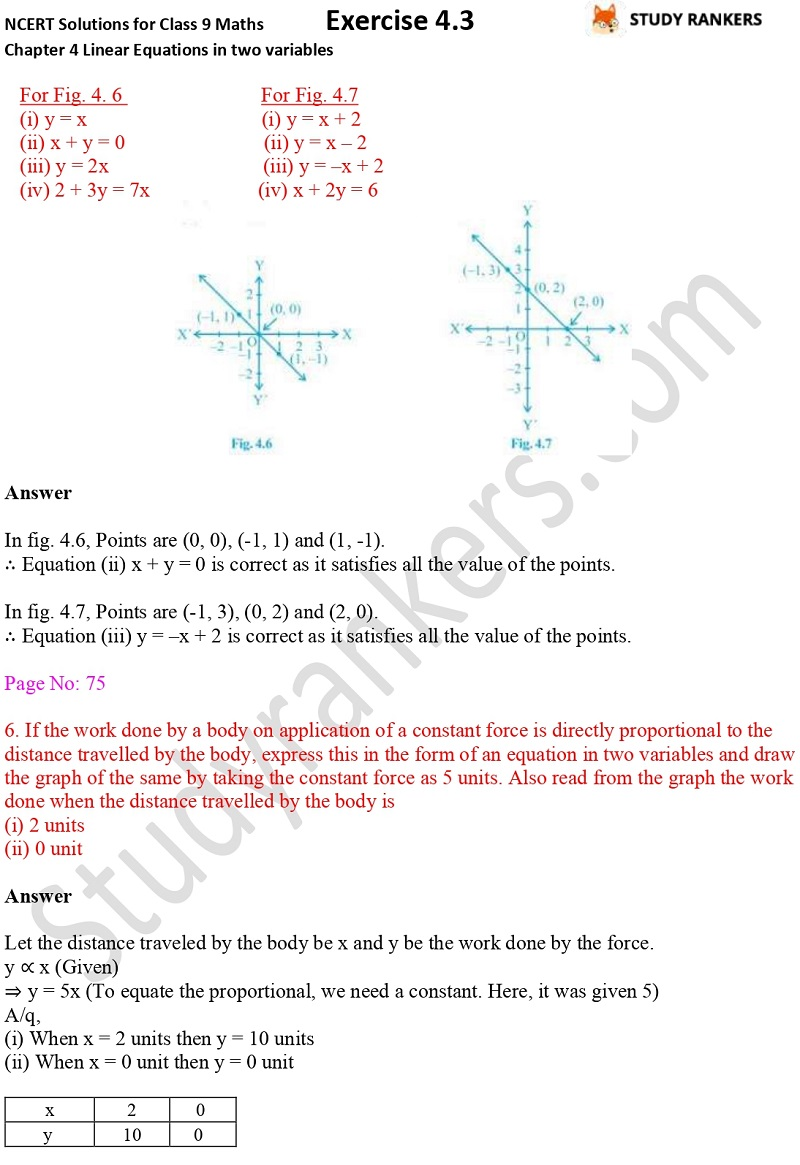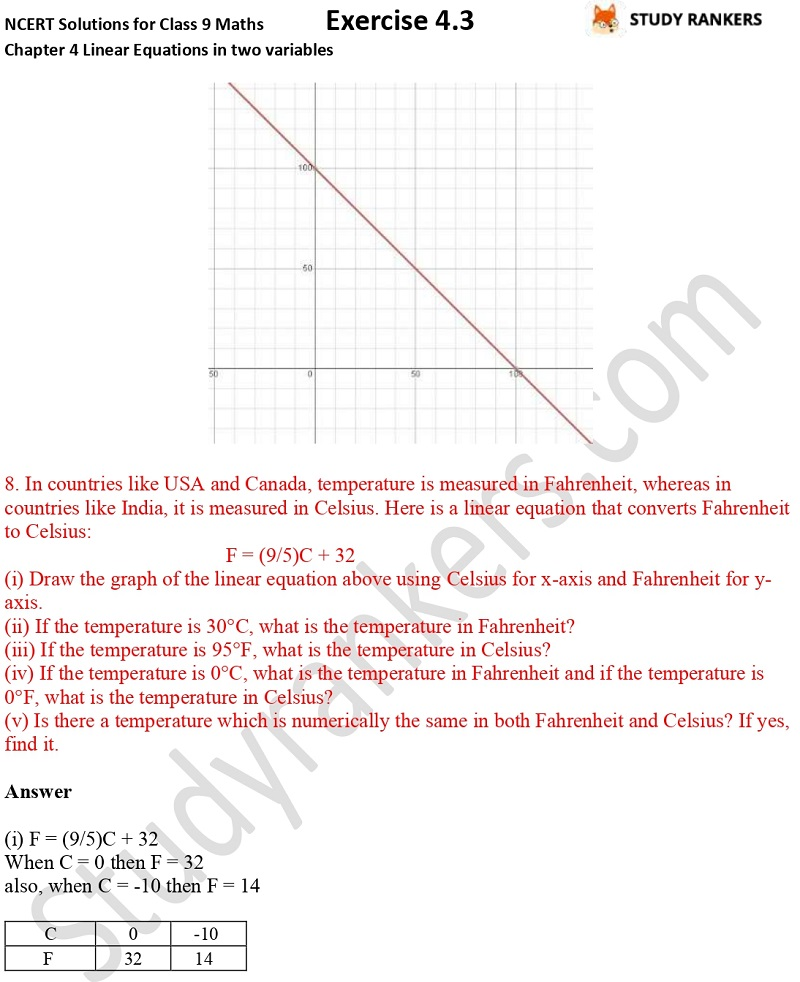## NCERT Solutions for Class 9 Maths Chapter 4 Linear Equations in Two Variables Exercise 4.3

Exercise 4.3 Chapter 4 Linear Equations in Two Variables NCERT Solutions for Class 9 Maths is very useful in finding the solutions of difficult questions. Class 9 Maths NCERT Solutions are helpful in finding important concepts of the chapter. These are prepared by Studyrankers experts so you can easily find questions and answers.

Exercise 4.3 has total 8 questions in which you have to draw the graph of each of the given linear equations in two variables, give the equations of two lines passing through (2, 14) and etc.